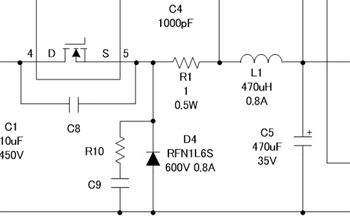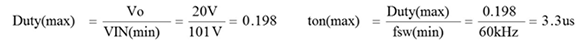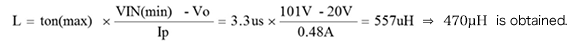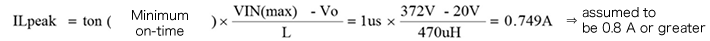Technical Information Site of Power Supply Design

2017.11.09 AC/DC

# Selecting Critical Components: Inductor L1

Design Examples of Non-isolated Buck Converters

In this second section on "Selecting Critical Components", we explain methods for selecting inductors, which play a vital role in switching power supplies.

Inductor L1

The inductor L1, together with the output capacitor C5, forms an LC filter, as indicated in the circuit diagram on the right. The inductance L1 is set so that the operating mode is a discontinuous mode. If the device is operated in a continuous mode, the reverse current that flows during the reverse recovery time trr of the diode causes diode losses to increase, and moreover the reverse current may reach the peak current while the MOSFET is ON, thus also increasing MOSFET losses. In order to avoid these increased losses, discontinuous mode is selected. For details please refer to the section “Basic Operation of Buck Converters and Discontinuous Mode vs. Continuous Mode”.Inductance Calculation

First, we calculate the inductance. We use 101 V for VIN. That is, we assume 90 VAC and use 1.41 times this value as the peak voltage, and add a 20% margin.

If VIN = 90VAC×1.41×0.8 = 101V, then:We add a 20% margin for Iomax, so that Iomax = 0.2 A×1.2 = 0.24 A.

If the critical point (peak) is Ip = Iomax×2 = 0.48 A, then:From this calculation result, we select 470 µH as the standard value.

Calculation of Inductor Current

Next, we calculate the inductor current, and determine the allowable current in the inductor.

The inductor current is maximum when the input voltage is maximum. The maximum input voltage is 264 VAC, and under this condition, the time during which the power supply IC turns the switch (MOSFET) on is the minimum on-time of the IC. This minimum on-time is approximately 0.6 to 1.5 µs, depending on the output voltage, the inductance of the inductor, and other parameters.

Taking 1 µs to be the minimum on-time when the maximum input voltage is 264 VAC:Hence the inductor that should be selected has an inductance of 470 μH and an inductor current of 0.8 A or greater. It should be remembered that the inductor current must always be checked in actual equipment, to confirm that the inductor does not reach saturation.

#### Key Points:

・The inductor should be set such that the operating mode is discontinuous mode.

・The inductance is determined from the VIN minimum condition and the maximum value of ton.

・The inductor current is determined from the VIN maximum condition and the minimum on-time.

This website uses cookies.

By continuing to browse this website without changing your web-browser cookie settings, you are agreeing to our use of cookies.